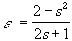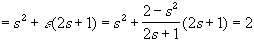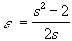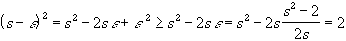#Interactive Real Analysis

Next | Previous | Glossary | Map

## 2.4. The Real Number System

### Theorem 2.4.5: Square Roots in R

There is a positive real number x such that x2 = 2

### Proof:

Since the above equation is not true in Q, we have to use a property of the real numbers that is not true for the rational numbers. Define the set
• S = { tR: t > 0 and t22 }
Our hope is that the supremum of this set should be the desired solution to the above equation. However, we first need to make sure that the supremum indeed exists before showing that it is the desired solution.

S is not empty, because 1 is contained in S, and S is bounded above by, say, 5. Hence, using the least upper bound property of the real numbers, S has a least upper bound s:

• s = sup(S)
Note that this would not necessarily be true if we restricted ourselves to the rational numbers.

Now we hope that s2 = 2, i.e. s is the desired solution. Since 1 is in S, we know

• s > 1
Now s either is the solution, or one of the following two cases are true:
Is s2 < 2 ?
Let. Then, by assumption 0 << 1, so thatHence, s +is also in S, in which case s can not be an upper bound for S. This is a contradiction, so this case is not possible.

Is s2 > 2 ?
Let. Again> 0, so thatHence, s -is another upper bound for S, so that s is not the least upper bound for S. This is a contradiction, so that this case is not possible.
Having eliminated these two cases, we are left with s2 = 2, which is what we wanted to prove.Next | Previous | Glossary | Map